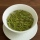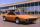# Vinegar mixing

We mix 70g water and 50g 8% vinegar. How many percents of the solution of vinegar we produce?

Result

p =  3.333 %

#### Solution:Leave us a comment of example and its solution (i.e. if it is still somewhat unclear...):

Showing 0 comments:Be the first to comment!#### To solve this example are needed these knowledge from mathematics:

Our percentage calculator will help you quickly calculate various typical tasks with percentages.

## Next similar examples:

1. Fruit teaTea contains 7% of fruit components and 12% of sugar in this component. How many percents of sugar is represented in the whole tea?
2. PersonsPersons surveyed:100 with result: Volleyball=15% Baseball=9% Sepak Takraw=8% Pingpong=8% Basketball=60% Find the average how many like Basketball and Volleyball. Please show your solution.
3. Frameworks is badCalculate how many percent will increase the length of an HTML document, if any ASCII character unnecessarily encoded as hexadecimal HTML entity composed of six characters (ampersand, grid #, x, two hex digits and the semicolon). Ie. space as: &#x20;
4. ExaminationThe class is 21 students. How many ways can choose two to examination?
5. ChordsHow many 4-tones chords (chord = at the same time sounding different tones) is possible to play within 7 tones?
6. TeamsHow many ways can divide 16 players into two teams of 8 member?
7. Percentage 1052 shorts and missed 13. Calculate percentage
8. SpeedometerThe speedometer of a car reads 66 km/h. It's accurate to within 4.1% What is the maximum possible error?
9. Profit gainIf 5% more is gained by selling an article for Rs. 350 than by selling it for Rs. 340, the cost of the article is:
10. BorrowingI borrow 25,000 to 6.9% p.a.. I pay 500 per month. How much will I pay and for how long?
11. LossA bookstore purchased from a publisher the biography of a well-known politician for R15 per copy, but sales have been very poor. The manager has decided to mark the copies down to R12 each to make a quick sale. Calculate the loss on each book as a percenta
12. SequenceFind the common ratio of the sequence -3, -1.5, -0.75, -0.375, -0.1875. Ratio write as decimal number rounded to tenth.
13. Theorem proveWe want to prove the sentense: If the natural number n is divisible by six, then n is divisible by three. From what assumption we started?
14. AP - simpleDetermine the first nine elements of sequence if a10 = -1 and d = 4
15. LegsCancer has 5 pairs of legs. The insect has 6 legs. 60 animals have a total of 500 legs. How much more are cancers than insects?
16. Three unknownsSolve the system of linear equations with three unknowns: A + B + C = 14 B - A - C = 4 2A - B + C = 0
17. AverageIf the average(arithmetic mean) of three numbers x,y,z is 50. What is the average of there numbers (3x +10), (3y +10), (3z+10) ?